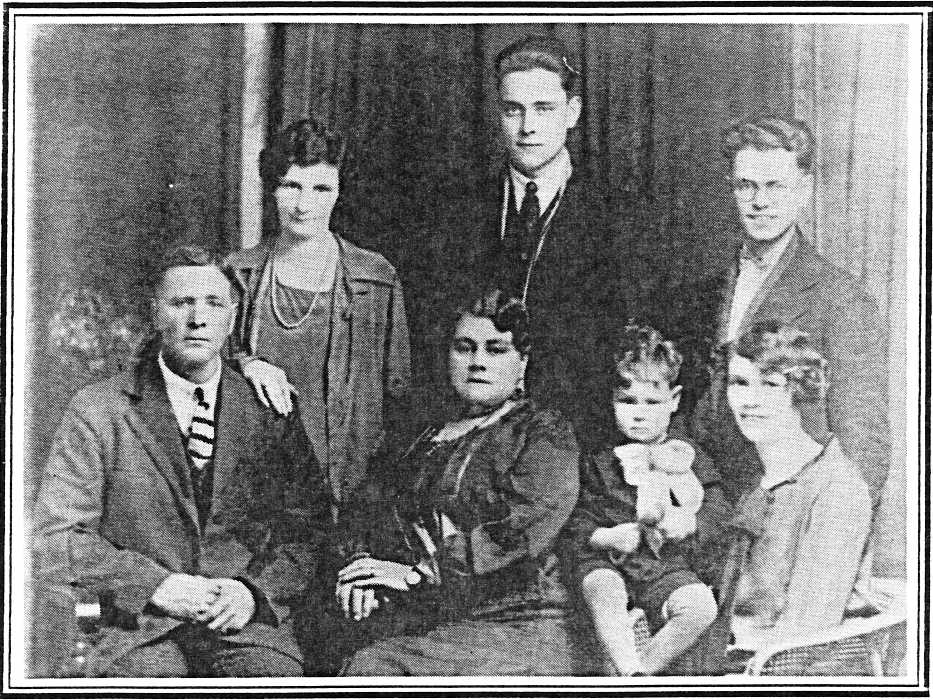## Family Photo

Place mouse over face for identification of personDescription Family Photo Date / Time 1927-00-00 Country / State / County / City / Placename CAN Persons (t-b,l-r) Ruth Mary (nee Lidgote) McBride, Orvil McBride, Earl Thomas McBride, Thomas James McBride, Annie Clark McBride, Raymond Orvil McBride, Evlyn Mabel (nee Dahl) McBride
```lib card:
dc:
title 		=	Family Photo
creator 	=	Jerome Goulet
subject 	=
description 	=	Original (scanned analog or digital), names to faces, stored to LibCard "format", within image exif:
publisher 	=	Jerome Goulet
contributor 	=
date 		=	1927-00-00
resourcetype 	=
iformat 	=
if_mime 	=	image/png
if_resolution 	=	72x72
if_dimensions 	=
if_dim_x 	=	933
if_dim_y 	=	700
resourceID 	=
source 		=	photograph (analog or digital)
language 	=	eng
rights 		=	Copyright 2020, Jerome Goulet<jtgoulet@yahoo.com>, GNU General Public License
lc:
when:
dateUTC		=	1927-00-00
timeUTC		=	0000
tz		=	+0000

dateLocal	=	1927-00-00
timeLocal	=	0000
where:
admin0		=	CAN
admin1		=	x
admin2		=	x
admin3		=	x
pName		=	x
lat		=	x
lon		=	x
who:
person:
name:
surName		=	McBride
givenName	=	Ruth Mary (nee Lidgote)
aka		=	RMM
relation	=
pip:	(person in picture)
boxX		=	267
boxY		=	158
boxWidth	=	78
boxHeight	=	78
label		=
uri		=
person:
name:
surName		=	McBride
givenName	=	Orvil
aka		=	OM
relation	=
pip:	(person in picture)
boxX		=	484
boxY		=	73
boxWidth	=	91
boxHeight	=	91
label		=
uri		=
person:
name:
surName		=	McBride
givenName	=	Earl Thomas
aka		=	ETM
relation	=
pip:	(person in picture)
boxX		=	719
boxY		=	152
boxWidth	=	84
boxHeight	=	84
label		=
uri		=
person:
name:
surName		=	McBride
givenName	=	Thomas James
aka		=	TJM
relation	=
pip:	(person in picture)
boxX		=	150
boxY		=	264
boxWidth	=	95
boxHeight	=	95
label		=
uri		=
person:
name:
surName		=	McBride
givenName	=	Annie Clark
aka		=	ACM
relation	=
pip:	(person in picture)
boxX		=	437
boxY		=	315
boxWidth	=	84
boxHeight	=	84
label		=
uri		=
person:
name:
surName		=	McBride
givenName	=	Raymond Orvil
aka		=	ROM
relation	=
pip:	(person in picture)
boxX		=	606
boxY		=	374
boxWidth	=	70
boxHeight	=	70
label		=
uri		=
person:
name:
surName		=	McBride
givenName	=	Evlyn Mabel (nee Dahl)
aka		=	EMM
relation	=
pip:	(person in picture)
boxX		=	712
boxY		=	353
boxWidth	=	81
boxHeight	=	81
label		=
uri		=
what:
eventName	=	Family Photo
```